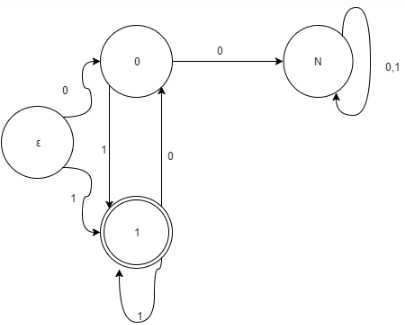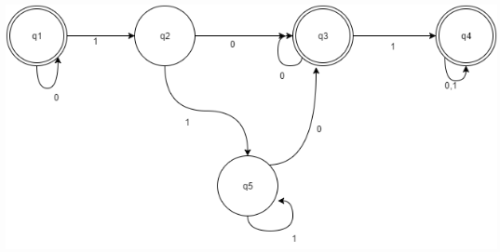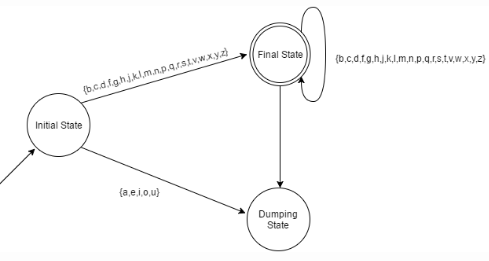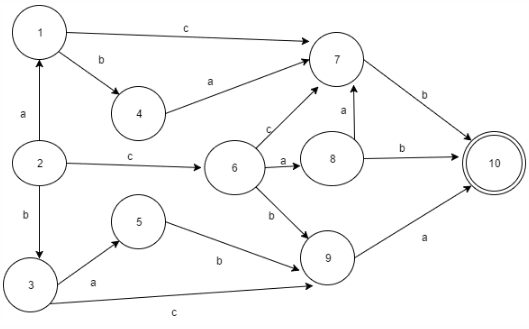Courses

# Test: DFA Processing Strings

## 9 Questions MCQ Test Theory of Computation | Test: DFA Processing Strings

Description
This mock test of Test: DFA Processing Strings for Computer Science Engineering (CSE) helps you for every Computer Science Engineering (CSE) entrance exam. This contains 9 Multiple Choice Questions for Computer Science Engineering (CSE) Test: DFA Processing Strings (mcq) to study with solutions a complete question bank. The solved questions answers in this Test: DFA Processing Strings quiz give you a good mix of easy questions and tough questions. Computer Science Engineering (CSE) students definitely take this Test: DFA Processing Strings exercise for a better result in the exam. You can find other Test: DFA Processing Strings extra questions, long questions & short questions for Computer Science Engineering (CSE) on EduRev as well by searching above.
QUESTION: 1

### The password to the admins account=”administrator”. The total number of states required to make a password-pass system using DFA would be __________

Solution:

For a string of n characters with no repetitive substrings, the number of states required to pass the string is n+1.

QUESTION: 2

### Which of the following is the corresponding Language to the given DFA?Solution:

The Language can be anonymously checked and thus the answer can be predicted. The language needs to be accepted by the automata (acceptance state) in order to prove its regularity.

QUESTION: 3

### Let ∑= {a, b, …. z} and A = {Hello, World}, B= {Input, Output}, then (A*∩B) U (B*∩A) can be represented as:

Solution:

Union operation creates the universal set by combining all the elements of first and second set while intersection operation creates a set of common elements of the first and the second state.

QUESTION: 4

Let the given DFA consist of x states. Find x-y such that y is the number of states on minimization of DFA?Solution:

Use the equivalence theorem or Myphill Nerode theorem to minimize the DFA

QUESTION: 5

For a machine to surpass all the letters of alphabet excluding vowels, how many number of states in DFA would be required?

Solution:QUESTION: 6

6. For the DFA given below compute the following:
Union of all possible combinations at state 7,8 and 9.Solution:

The string a state receives is the combination of all input alphabets which lie across the path covered.

QUESTION: 7

Given L= {Xϵ∑*= {a, b} |x has equal number of a, s and b’s}.
Which of the following property satisfy the regularity of the given language?

Solution:

DFA can be made for infinite language with an infinite length. Thus, dependency over length is unfruitful.

QUESTION: 8

Given:
L= {xϵ∑= {0,1} |x=0n1n for n>=1}; Can there be a DFA possible for the language?

Solution:

It is not possible to have a count of equal number of 0 and 1 at any instant in DFA. Thus, It is not possible to build a DFA for the given Language.

QUESTION: 9

The password to the admins account=”administrator”. The total number of states required to make a password-pass system using DFA would be __________

Solution:

For a string of n characters with no repetitive substrings, the number of states required to pass the string is n+1.